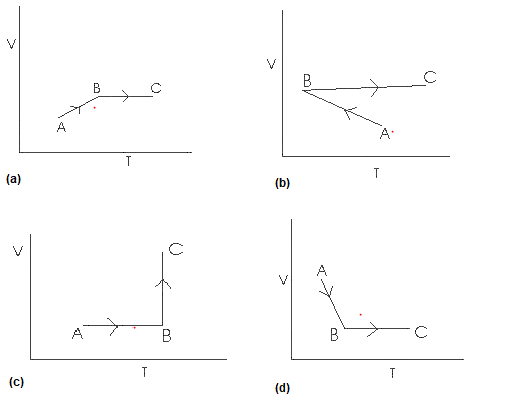# P-V Diagram Problems and Solutions

In this page we have P-V Diagram Problems and Solutions on heat and thermodynamics for JEE and Competitive examinations . Hope you like them and do not forget to like , social share and comment at the end of the page.

Question 1
A cyclic Process ABCA as shown in below V-T diagram is performed with a constant mass of ideal gas.Show the process in the P-V digram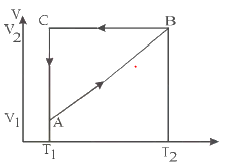Question 2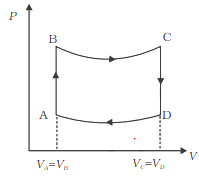A cycle followed by an engine (made of one mole of perfect gas in a cylinder with a piston) as shown above
A to B : volume constant
C to D : volume constant
(a) In which part of the cycle heat is supplied to the engine from outside?
(b) In which part of the cycle heat is being given to the surrounding by the engine?

Question 3:
The internal energy of an ideal gas depends on
(a) Temperature
(b) Pressure
(c) Volume
(d) None of these

Question 4
The below are P-V diagrams for cyclic processes for a gas.Which of the below processes heat is absorbed by the gas?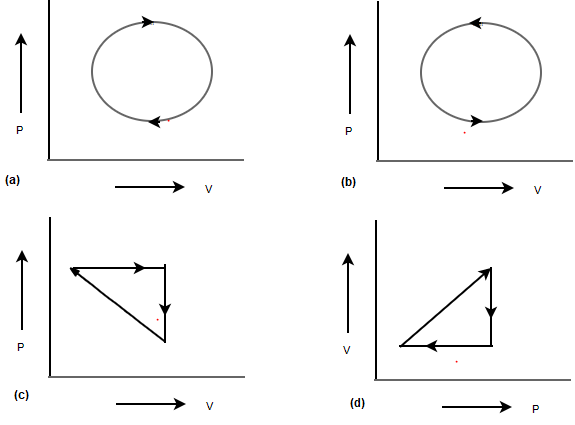Question 5
An ideal gas is taken through a cyclic thermodynamics process through four steps.
The amount of heat involved in the steps are Q1 = 5960 J,Q2 = - 5600 J,Q3= -3000 J,Q4 = -3600 J respectively. The corresponding quantities of Internal energy changes are ΔU1 = 3.760 J ,ΔU2 = - 4800 J,ΔU3 = -1800 J,ΔU4 = ?
find the value ΔU4 & net work done
(a) 2930 J, 960 J
(b) 2830 J, 900 J
(c) 2930 J, -960 J
(d) -2930 J, 960 J

Question 6
4 moles of an ideal gas undergoes an isothermal expansion at temperature T during which the volume becomes $\eta$ times.
W -> Work done by the gas
$\Delta U$ -> Change in internal energy
(a) $W=-4RT ln \eta$
$\Delta U =0$
(b) $W=-RT ln \eta$
$\Delta U =\frac {6RT}{5}$
(c) $W=RT ln \eta$
$\Delta U =\frac {6RT}{5}$
(d) $W=4RT ln \eta$
$\Delta U =0$

Question 7
A cyclic Process is shown in the given below p-T digram. Which of the following curves shows the same process in p-V diagram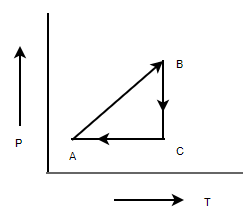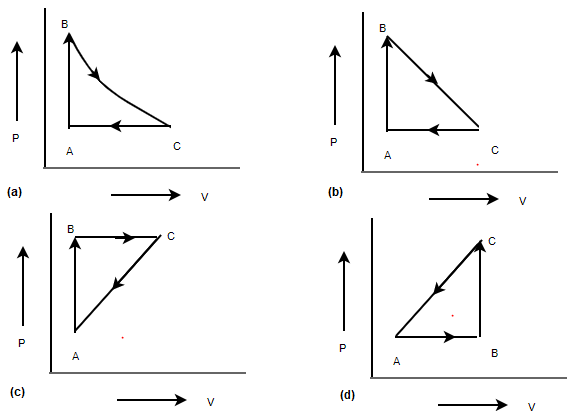Question 8
A cyclic Process is shown in the given below p-T digram. Which of the following curves shows the same process in V-T diagram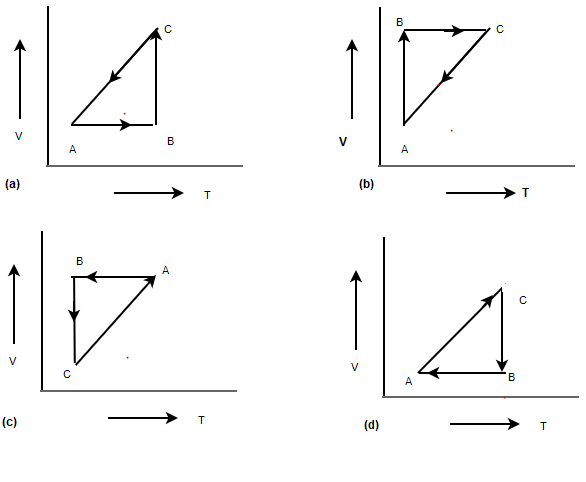Question 9
A cyclic Process is shown in the given below p-T digram.Here AC is an adiabatic Process Which of the following curves shows the same process in P-V diagram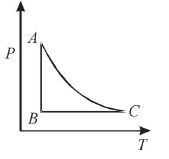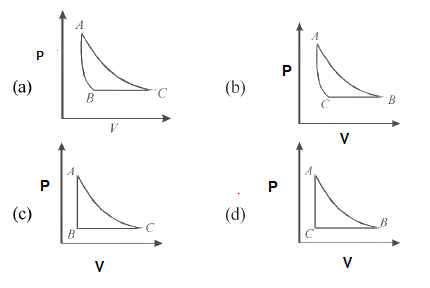Question 10
n moles of an ideal gas undergoes an process A to B as shown in the below figure. The maximum temperature of the gas during the process is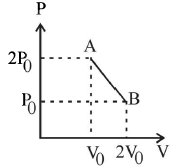(a) $\frac {9P_0V_0}{2nR}$
(b) $\frac {9P_0V_0}{nR}$
(c) $\frac {9P_0V_0}{4nR}$
(d) $\frac {3P_0V_0}{2nR}$

Question 11
A system goes from state A to B via path I and II
$\Delta U_1$ -> Change in internal energy in Path I
$\Delta U_2$ -> Change in internal energy in Path II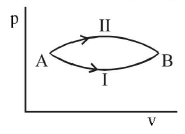Which of the following is true?
(a) $\Delta U_1 > \Delta U_2$
(b) $\Delta U_1 < \Delta U_2$
(c) $\Delta U_1 = \Delta U_2$
(d) Relation between $\Delta U_1$ and $\Delta U_2$ cannot be determined

Question 12
Match the Column I to Column II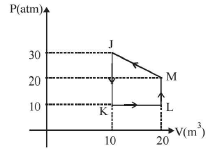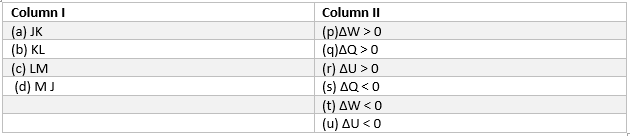Question 13
An ideal gas under goes an process show in P-V diagram.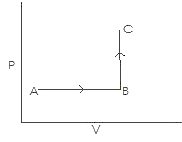Which of the diagram represent it on V-T diagram